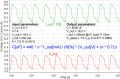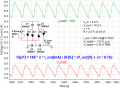#### Ghina Bayyat

Joined Mar 11, 2018
123
i have a problem on determining the appropriate value of the capacitors in a voltage multiplier circuit [ what ever it is full or half wave ]
is there a specific low to determine their values ??
also is it true that a circuit with high input frequency needs capacitors with small capacitance and for low input frequency capacitors with high capacitance are required ??

#### MrChips

Joined Oct 2, 2009
19,923
True. However, the value of the capacitor will determine how much charge is transferred and hence how much current the multiplier can supply.

Joined Mar 10, 2018
3,791

#### Ghina Bayyat

Joined Mar 11, 2018
123
True. However, the value of the capacitor will determine how much charge is transferred and hence how much current the multiplier can supply.
u mean its capacitance isn't related to the voltage but to the current ?
and isn't there an equation to determine it ? for example
i have a voltage multiplier circuit with Vin = 4.8v and f = 50 hz
i wanna multiply it 4 times to become 19.2v and the current is 250mA so how can i determine the value of the four capacitors ??

#### -live wire-

Joined Dec 22, 2017
901
Why not use a step-up transformer? Pin ~ Pout, so if your low voltage can supply enough current, then you can draw some decent current on the output.

#### Danko

Joined Nov 22, 2017
851
Simplified formula for 50Hz: С[uF] = 2,85 * n * I_out[mA] / (K * V_out[V]),
where n - multiplying coefficient (4 in your case),
K - ripples value[%].

Last edited:

#### Ghina Bayyat

Joined Mar 11, 2018
123
surely it's so important but didn't find what i was looking for

Why not use a step-up transformer? Pin ~ Pout, so if your low voltage can supply enough current, then you can draw some decent current on the output.
it's good of course but costs almost more than the whole device i'm working at
it's a simple device so no need for it thank u

Danko said:
Simplified formula for 50Hz: С[uF] = 2,85 * n * I_out[mA] / (K * V_out[V]),
where n - multiplying coefficient (4 in your case),
K - ripples value[%].
i'd like to know where did u get this formula from ? i was looking for something like this but didn't find it anywhere
sorry to ask u this but are u sure of it because u are the first person who gives me an equation for it and lots of sites and references said that it's only known by experience

Last edited by a moderator:

#### -live wire-

Joined Dec 22, 2017
901
Here is a good general formula:

Q = C*R

Quality = cost times reliability. This is just an equation I have learned from experience.

#### Danko

Joined Nov 22, 2017
851
•Bernard

#### Ghina Bayyat

Joined Mar 11, 2018
123
Here is a good general formula:

Q = C*R

Quality = cost times reliability. This is just an equation I have learned from experience.
thanks it's a very important advice i appreciate it

On site http://elektricvdome.ru/umnozhitel-napryazheniya/
they have typo. Current should be not in mA, but in uA.
So, rigt formula is:
С[uF] = 2,85 * n * I_out[uA] / (K * V_out[V])
i tried the equation with i out in mA and the value of c i got is 28 nf and i tried this value in simulation but in practice i tried a capacitor with a value of 1uf and the result for both values was perfect [ without a load ] and i got Vrip = 50mv only but with a load of 1k ohms resistor i had to use a capacitor of 1000uf to get Vrip = 0.5v
but if as u said i try it with iout in uA the result is 28000uf and this can't be a right value for a simple circuit like this
I simulated your multiplier for 0.1% ripples:
i'm used to consider K = 2.5 % as a practice value
and also in ur simulation the capacitors value is too big i think it's more right with iout in mA

Last edited by a moderator:
•-live wire-

#### Danko

Joined Nov 22, 2017
851
i'm used to consider K = 2.5 % as a practice value
and also in ur simulation the capacitors value is too big i think it's more right with iout in mA
You are right, formula from website is not for uA, but it is not for mA tooHere is enhanced, more accurate formula for series multiplier, also takes in account voltage drops on diodes:
C[uF] =440 * n * I_out[mA] / (K[%] * (V_out[V] + (n * 0.7)))
With your input parameters 4,8V, 19.2V, 250mA, K=2.5%(0.48V) it works very good.
Only K is a little bit higher. See simulation result:Edit:
Here is formula for parallel multiplier circuit, better parameters, lower capacitors (3000uF instead 8000uF at 250mA output current):
C[uF] =165 * n * I_out[mA] / (K[%] * (V_out[V] + (n * 0.7)))Last edited:

#### Ghina Bayyat

Joined Mar 11, 2018
123
You are right, formula from website is not for uA, but it is not for mA tooHere is enhanced, more accurate formula for series multiplier, also takes in account voltage drops on diodes:
C[uF] =440 * n * I_out[mA] / (K[%] * (V_out[V] + (n * 0.7)))
With your input parameters 4,8V, 19.2V, 250mA, K=2.5%(0.48V) it works very good.
Only K is a little bit higher. See simulation result:
View attachment 150229
Edit:
Here is formula for parallel multiplier circuit, better parameters, lower capacitors (3000uF instead 8000uF at 250mA output current):
C[uF] =165 * n * I_out[mA] / (K[%] * (V_out[V] + (n * 0.7)))
View attachment 150248
yes but since i got a perfect value for Vout and Vrip and a low value for the capacitor why not to use it ?
after all those equations are for different companies to make their own units of voltage multipliers
of course i can use them and they will help me but it's not that i have to only depend on them i can change the values as i wish and i think the values i got before are good
but i have a question is K= 2.5 % a big value ?
i was told once that it's a good value to use in practice

C[uF] =440 * n * I_out[mA] / (K[%] * (V_out[V] + (n * 0.7)))
With your input parameters 4,8V, 19.2V, 250mA, K=2.5%(0.48V) it works very good.
Only K is a little bit higher. See simulation result:Edit:
also how did u get c = 8000uf ? it's supposed to be 8uf
because
c = 440 * n * 0.25 / (2.5 * (19.2 + 0.7 *4)
c = 440 / 55 = 8 and the unit is in uf right ?
or did i miss something because i usually forget lots of things and jump in solutions

Last edited by a moderator:

#### Danko

Joined Nov 22, 2017
851
yes but since i got a perfect value for Vout and Vrip and a low value for the capacitor why not to use it ?
You can do everything you wish, but in your first post #1 you asked: "i have a problem on determining the appropriate value of the capacitors in a voltage multiplier circuit [ what ever it is full or half wave ] is there a specific low to determine their values ??".
Since formula from website http://elektricvdome.ru/umnozhitel-napryazheniya/ is not correct, I rebuild it for both cases - series and parallel multipliers, because I bear responsibility for my answer. Nothing more.
after all those equations are for different companies to make their own units of voltage multipliers of course i can use them and they will help me but it's not that i have to only depend on them i can change the values as i wish and i think the values i got before are good
Yes, you can, and even you should, for practice improvement.
but i have a question is K= 2.5 % a big value ?
i was told once that it's a good value to use in practice
With K=2.5%, value of capacitance is moderately low and such level of ripple allows to feed LEDs, relays, some of digital logic circuits.
And you can use voltage regulator, for example LM7812, and get stabilized 12V with very small ripple, from 19.2V.
also how did u get c = 8000uf ? it's supposed to be 8uf
because
c = 440 * n * 0.25 / (2.5 * (19.2 + 0.7 *4)
c = 440 / 55 = 8 and the unit is in uf right ?
or did i miss something because i usually forget lots of things and jump in solutions
Your calculation for 0.25mA is right: C = 8uF.
But, I think, you typed 0.25[A] instead 250[mA].

Last edited:

#### Ghina Bayyat

Joined Mar 11, 2018
123
You can do everything you wish, but in your first post #1 you asked: "i have a problem on determining the appropriate value of the capacitors in a voltage multiplier circuit [ what ever it is full or half wave ] is there a specific low to determine their values ??".
Since formula from website http://elektricvdome.ru/umnozhitel-napryazheniya/ is not correct, I rebuild it for both cases - series and parallel multipliers, because I bear responsibility for my answer. Nothing more.

Yes, you can, and even you should, for practice improvement.

With K=2.5%, value of capacitance is moderately low and such level of ripple allows to feed LEDs, relays, some of digital logic circuits.
And you can use voltage regulator, for example LM7812, and get stabilized 12V with very small ripple, from 19.2V.

Your calculation for 0.25mA is right: C = 8uF.
But, I think, you typed 0.25[A] instead 250[mA].
thank u for ur help i could do it perfectly